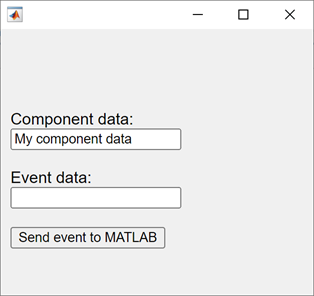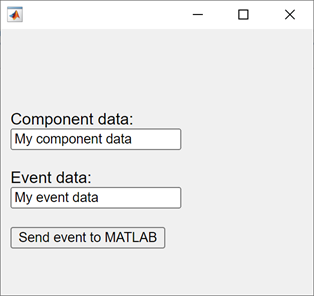## Create HTML Content in Apps

You can add HTML content, including JavaScript®, CSS, and third-party visualizations or widgets, to your app by using an HTML UI component. Use the `uihtml` function to create an HTML UI component.

When you add an HTML UI component to your app, write code to communicate between MATLAB® and JavaScript. You can set component data, respond to changes in data, and react to user interaction by sending events.

### Communicate Between MATLAB and JavaScript

To connect the MATLAB HTML UI component in your app to your HTML content, implement a `setup` function in your HTML file. The `setup` function defines and initializes a local JavaScript `htmlComponent` object, which synchronizes with the MATLAB `HTML` object. The JavaScript `htmlComponent` object is accessible only from within the `setup` function.

The `setup` function executes whenever one of these events happens:

• The HTML UI component is created in the UI figure and the content has fully loaded.

• The `HTMLSource` property of the MATLAB `HTML` object changes to a new value.

With this connection, you can share information between your MATLAB and JavaScript code using multiple approaches:

• Share component data — Use this approach when your HTML component has static data that you need to access from both your MATLAB and JavaScript code. For example, if your component contains a table, store the table data as shared component data.

• Send component events — Use this approach to broadcast a notification of a change or interaction. You can send an event from JavaScript to MATLAB or from MATLAB to JavaScript. For example, send an event from JavaScript when a user clicks a button HTML element to react to this interaction in your MATLAB code.

This table gives an overview of the ways that the MATLAB `HTML` object and the JavaScript `htmlComponent` object can communicate.

Access component object.

MATLAB represents the UI component as an `HTML` object.

You can access the `HTML` object from MATLAB by storing the output of the `uihtml` function as a variable.

```fig = uifigure; c = uihtml(fig);```

JavaScript represents the UI component as the `htmlComponent` object.

You can access the `htmlComponent` object only from within the `setup` function of the HTML source file associated with the MATLAB `HTML` object.

```<script type="text/javascript"> function setup(htmlComponent) { // Access the htmlComponent object here } </script> ```

Access component data.

The MATLAB `HTML` object has a `Data` property. This property is synchronized with the `Data` property of the JavaScript `htmlComponent` object. Use this property to transfer data to or access data from your HTML source code.

Access the property in your MATLAB code.

```fig = uifigure; c = uihtml(fig); c.Data = 10;```

The JavaScript `htmlComponent` object has a `Data` property. This property is synchronized with the `Data` property of the MATLAB `HTML` object. Use this property to transfer data to or access data from MATLAB.

Access the property in the `setup` function of the HTML source file.

```<script type="text/javascript"> function setup(htmlComponent) { htmlComponent.Data = 5; } </script> ```

Respond to a change in component data.

The MATLAB `HTML` object has a `DataChangedFcn` callback property.

Create a `DataChangedFcn` callback for the `HTML` UI component to execute a function when the `Data` property of the `htmlComponent` JavaScript object changes.

```fig = uifigure; c = uihtml(fig); c.DataChangedFcn = @(src,event) disp(event.Data);```

The JavaScript `htmlComponent` object has an `addEventListener` method.

Add a `DataChanged` listener to the `htmlComponent` object to execute a function when the `Data` property of the MATLAB `HTML` object changes.

```htmlComponent.addEventListener("DataChanged", updateData); function updateData(event) { let changedData = htmlComponent.Data; // Update HTML or JavaScript with the new data }```

For more information about the `addEventListener` method, see EventTarget.addEventListener() on Mozilla® MDN web docs.

Send and react to an event from MATLAB in JavaScript.

To send an event from MATLAB to JavaScript, use the `sendEventToHTMLSource` function.

For example, you can send an event to react to a user interacting with a MATLAB UI component in your JavaScript code.

```fig = uifigure; c = uihtml(fig); eventData = [1 2 3]; sendEventToHTMLSource(c,"myMATLABEvent",eventData);```

To react to an event from MATLAB in your JavaScript code, add a listener that listens for the MATLAB event to the JavaScript `htmlComponent` object.

Access event data sent from MATLAB using the `event.Data` property.

```htmlComponent.addEventListener("myMATLABEvent", processEvent); function processEvent(event) { let eventData = event.Data; // Update HTML or JavaScript to react to the event }```
Send and react to an event from JavaScript in MATLAB.

To react to an event from JavaScript in your MATLAB code, create an `HTMLEventReceivedFcn` callback for your `HTML` UI component.

```fig = uifigure; c = uihtml(fig); c.HTMLEventReceivedFcn = @(src,event) disp(event);```

To send an event from JavaScript to MATLAB, use the `sendEventToMATLAB` function.

For example, you can send an event to react to a user clicking an HTML button element in your MATLAB code.

```eventData = [1,2,3]; htmlComponent.sendEventToMATLAB("myHTMLEvent",eventData);```

For an example of an HTML source file that is configured to connect to a MATLAB `HTML` UI component, see Sample HTML Source File.

### Convert Data Between MATLAB and JavaScript

You can pass two types of data between the MATLAB HTML component and the JavaScript `htmlComponent` object:

• Component data, stored in the `Data` property of each object

• Event data, associated with an event sent from MATLAB to JavaScript or JavaScript to MATLAB

Because MATLAB and JavaScript support slightly different sets of data types, the component converts the data when it is shared.

When the component converts data from MATLAB to JavaScript:

1. The component encodes the MATLAB data as JSON-formatted text using the `jsonencode` function.

2. The component parses the JSON-formatted text to JavaScript data using JSON.parse().

When the component converts data from JavaScript to MATLAB:

1. The component encodes the JavaScript data as JSON-formatted text using JSON.stringify().

2. The component parses the JSON-formatted text to MATLAB data using the `jsondecode` function.

### Sample HTML Source File

This example provides a sample HTML source file. Save this code to a file named `sampleHTMLFile.html`. You can use this sample file as a starting point for your own HTML UI components, or to explore how a component sends data between MATLAB and JavaScript.

The sample file creates three elements:

• An edit field to display and edit component data

• An edit field to display and edit event data

• A button to send an event from JavaScript to MATLAB

The `setup` function in the sample file defines four callback functions:

• `dataFromMATLABToHTML` — Update the Component data edit field with the current data. This function executes whenever the `Data` property of the MATLAB `HTML` object changes.

• `eventFromMATLABToHTML` — Update the Event data edit field with the data from the most recent event. This function executes whenever MATLAB sends an event named `"MyMATLABEvent"` to the HTML source.

• `dataFromHTMLToMATLAB` — Update the `Data` property of the JavaScript `htmlComponent` object with the text in the Component data edit field. This function executes whenever a user enters a new value in the edit field. The function triggers the `DataChangedFcn` callback of the MATLAB `HTML` object.

• `eventFromHTMLToMATLAB` — Send an event named `"MyHTMLSourceEvent"` with data from the text in the Event data edit field. This function executes whenever a user clicks the button. The function triggers the `HTMLEventReceivedFcn` callback of the MATLAB `HTML` object.

```<!DOCTYPE html> <html> <head> <script type="text/javascript"> function setup(htmlComponent) { console.log("Setup called:", htmlComponent); // Code response to data changes in MATLAB htmlComponent.addEventListener("DataChanged", dataFromMATLABToHTML); // Code response to events from MATLAB htmlComponent.addEventListener("MyMATLABEvent", eventFromMATLABToHTML); // Update the Data property of the htmlComponent object // This action also updates the Data property of the MATLAB HTML object // and triggers the DataChangedFcn callback function let dataInput = document.getElementById("compdata") dataInput.addEventListener("change", dataFromHTMLToMATLAB); // Send an event to MATLAB and trigger // the HTMLEventReceivedFcn callback function let eventButton = document.getElementById("send"); eventButton.addEventListener("click", eventFromHTMLToMATLAB); function dataFromMATLABToHTML(event) { let changedData = htmlComponent.Data; console.log("New data from MATLAB:", changedData); // Update your HTML or JavaScript with the new data let dom = document.getElementById("compdata"); dom.value = changedData; } function eventFromMATLABToHTML(event) { let eventData = event.Data; console.log("Event from MATLAB with event data:", eventData); // Update your HTML or JavaScript to react to the event let dom = document.getElementById("evtdata"); dom.value = eventData; } function dataFromHTMLToMATLAB(event) { newData = event.target.value; htmlComponent.Data = newData; console.log("New data in HTML:", newData) } function eventFromHTMLToMATLAB(event) { eventData = document.getElementById("evtdata").value; htmlComponent.sendEventToMATLAB("MyHTMLSourceEvent", eventData); console.log("Sending event to MATLAB with event data:", eventData); } } </script> </head> <body> <div style="font-family:sans-serif;"> <label for="compdata">Component data:</label> <input type="text" id="compdata" name="compdata"><br><br> <label for="evtdata">Event data:</label> <input type="text" id="evtdata" name="evtdata"><br><br> <button id="send">Send event to MATLAB</button> </div> </body> </html>```

#### Send Data and Events Between MATLAB and JavaScript

In MATLAB, create an HTML UI component and specify the HTML source as `sampleHTMLFile.html`. Assign `DataChangedFcn` and `HTMLEventReceivedFcn` callbacks that display the component data and event data, respectively.

```fig = uifigure; h = uihtml(fig, ... "HTMLSource","sampleHTMLFile.html", ... "DataChangedFcn",@(src,event) disp(src.Data), ... "HTMLEventReceivedFcn",@(src,event) disp(event.HTMLEventData), ... "Position",[20 20 200 200]);```

Specify the component `Data` property in MATLAB. The Component data field updates to display the data.

`h.Data = "My component data";`Send an event from MATLAB to JavaScript and specify some event data. The Event data field updates to display the data.

`sendEventToHTMLSource(h,"MyMATLABEvent","My event data")`Update the text in the Component data field, then press Enter. In MATLAB, the `DataChangedFcn` callback executes and displays the updated text in the Command Window.

Finally, update the text in the Event data field, then click the button. In MATLAB, the `HTMLEventReceivedFcn` callback executes and displays the updated text in the Command Window.